How to Calculate and Solve for Bulk Modulus of Elasticity (stress) | Rock Mechanics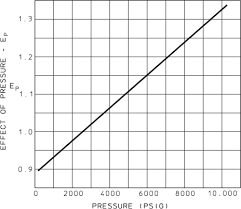The image above represents bulk modulus of elasticity (stress).

To compute for bulk modulus elasticity (stress), two essential parameters are needed and these parameters are Average Horizontal Stress (hav) and Vertical Stress (σv).

The formula for calculating the bulk modulus elasticity (stress):

k = hav / σv

Where;

k = Bulk Modulus of Elasticity (Stress)
hav = Average Horizontal Stress
σv = Vertical Stress

Let’s solve an example;
Given that the average horizontal stress is 15 and the vertical stress is 34. Find the bulk modulus of elasticity (stress)?

This implies that;

hav = Average Horizontal Stress = 15
σv = Vertical Stress = 34

k = hav / σv
k = 15 / 34
k = 0.44

Therefore, the bulk modulus of elasticity (stress) is 0.44.

Calculating the Average Horizontal Stress when the Bulk Modulus Elasticity (stress) and the Vertical Stress is Given.

hav = k x σv

Where;

hav = Average Horizontal Stress
k = Bulk Modulus of Elasticity (Stress)
σv = Vertical Stress

Let’s solve an example;
Given that the bulk modulus of elasticity (stress) is 25 and the vertical stress is 8. Find the average horizontal stress?

This implies that;

k = Bulk Modulus of Elasticity (Stress) = 25
σv = Vertical Stress = 8

hav = k x σv
hav = 25 x 8
hav = 200

Therefore, the average horizontal stress is 200.

Calculating the Vertical Stress when the Bulk Modulus Elasticity (stress) and the Average Horizontal Stress is Given.

σvhav / k

Where;

σv = Vertical Stress
k = Bulk Modulus of Elasticity (Stress)
hav = Average Horizontal Stress

Let’s solve an example;
Given that the bulk modulus of elasticity (stress) is 18 and the average horizontal stress is 32. Find the vertical stress?

This implies that;

k = Bulk Modulus of Elasticity (Stress) = 18
hav = Average Horizontal Stress = 32

σvhav / k
σv32 / 18
σv = 1.77

Therefore, the vertical stress is 1.77.

Nickzom Calculator – The Calculator Encyclopedia is capable of calculating the bulk modulus of elasticity (stress).

To get the answer and workings of the bulk modulus of elasticity (stress) using the Nickzom Calculator – The Calculator Encyclopedia. First, you need to obtain the app.

You can get this app via any of these means:

To get access to the professional version via web, you need to register and subscribe for NGN 1,500 per annum to have utter access to all functionalities.
You can also try the demo version via https://www.nickzom.org/calculator

Android (Paid) – https://play.google.com/store/apps/details?id=org.nickzom.nickzomcalculator
Android (Free) – https://play.google.com/store/apps/details?id=com.nickzom.nickzomcalculator
Apple (Paid) – https://itunes.apple.com/us/app/nickzom-calculator/id1331162702?mt=8
Once, you have obtained the calculator encyclopedia app, proceed to the Calculator Map, then click on Geology under Add-on.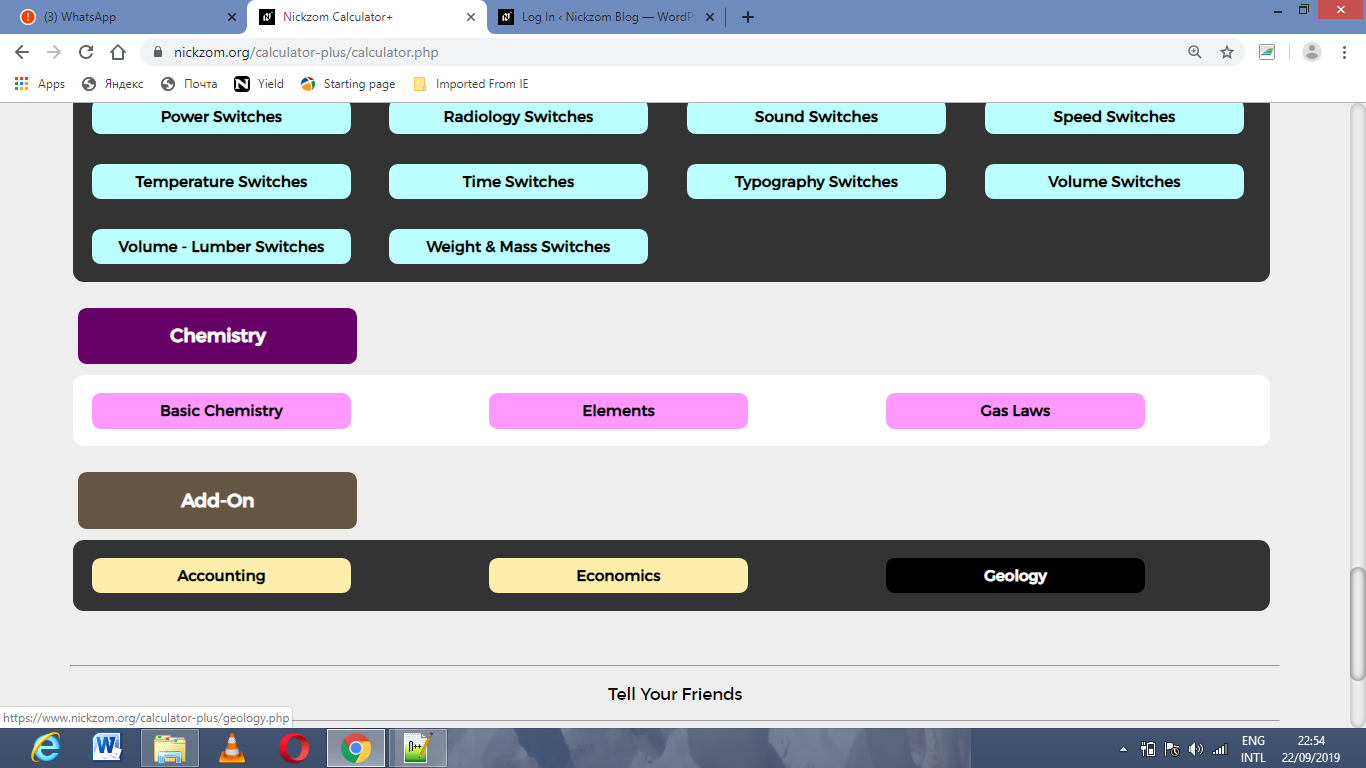Now, Click on Rock Mechanics under Geology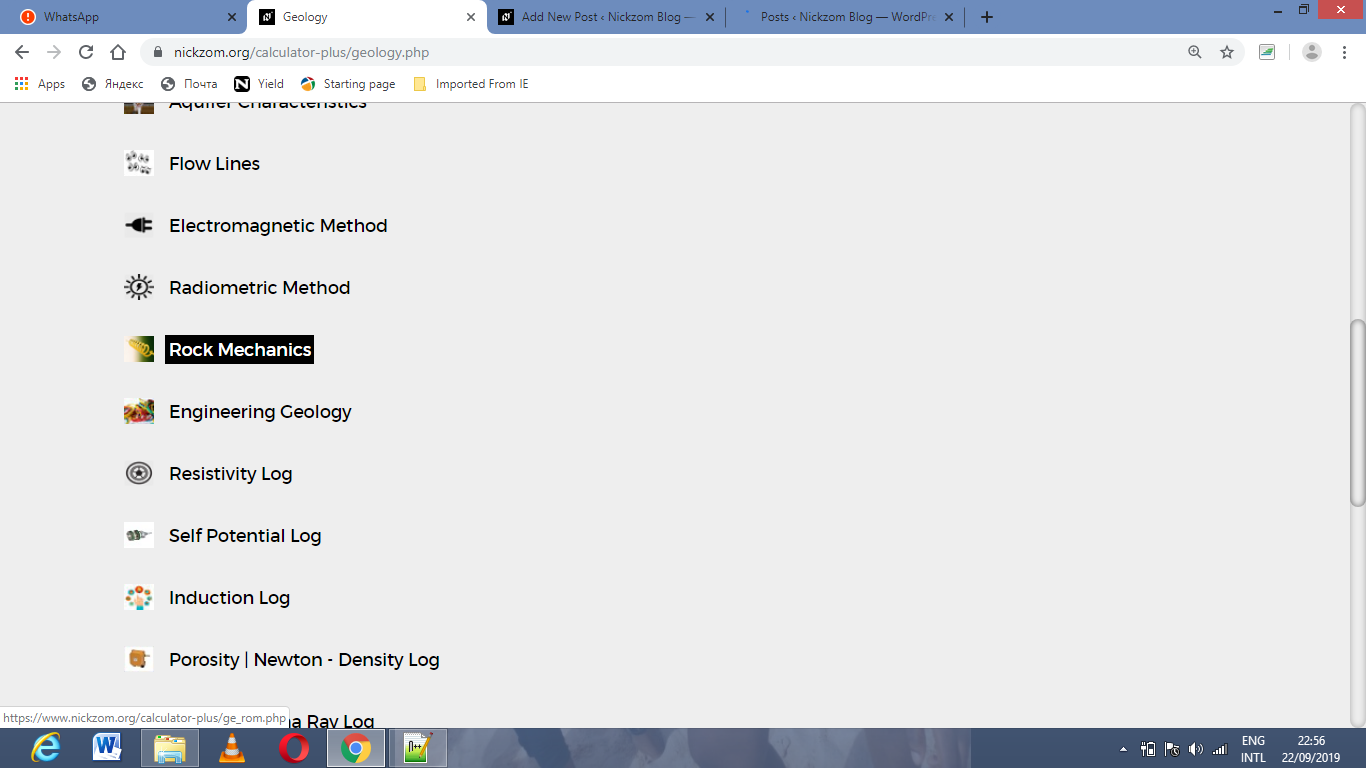Now, Click on Bulk Modulus of Elasticity (stress) under Rock Mechanics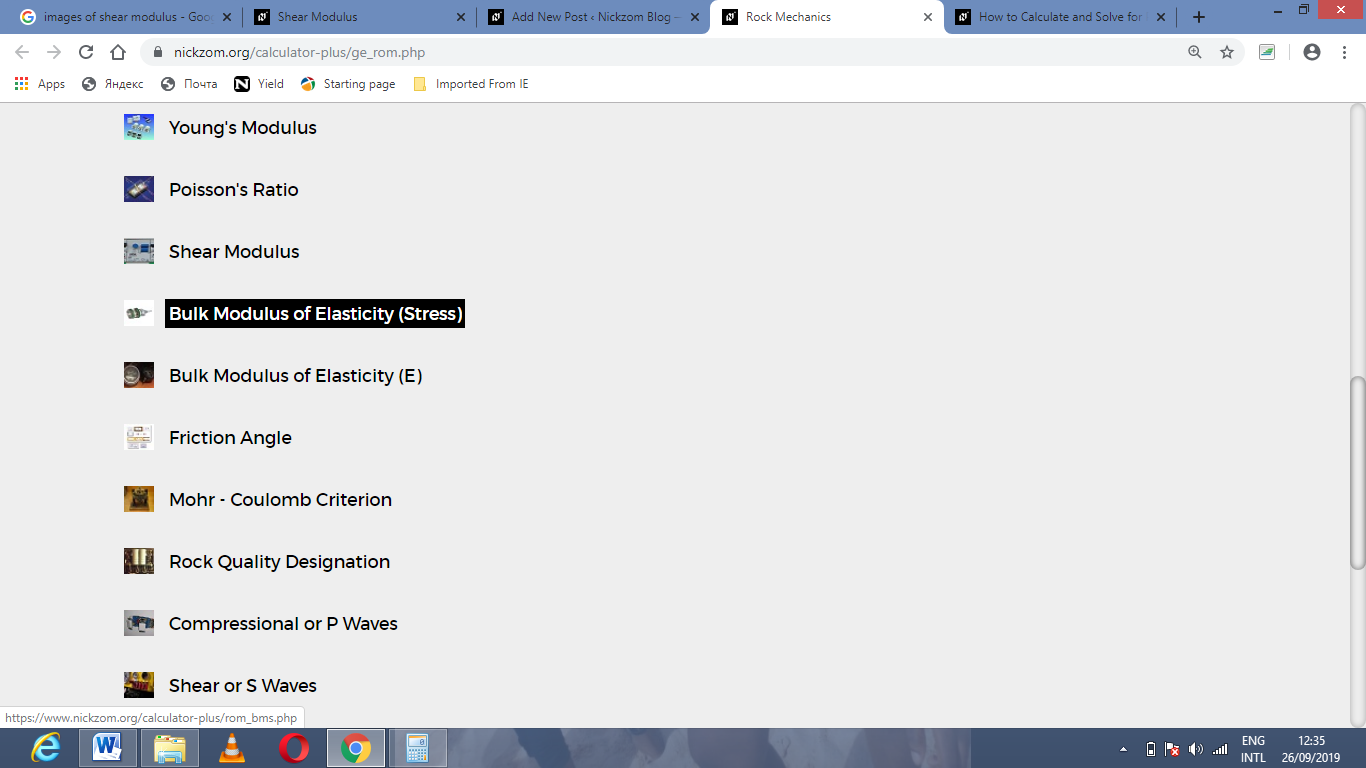The screenshot below displays the page or activity to enter your values, to get the answer for the bulk modulus of elasticity (stress) according to the respective parameters which are the Average Horizontal Stress (hav) and Vertical Stress (σv).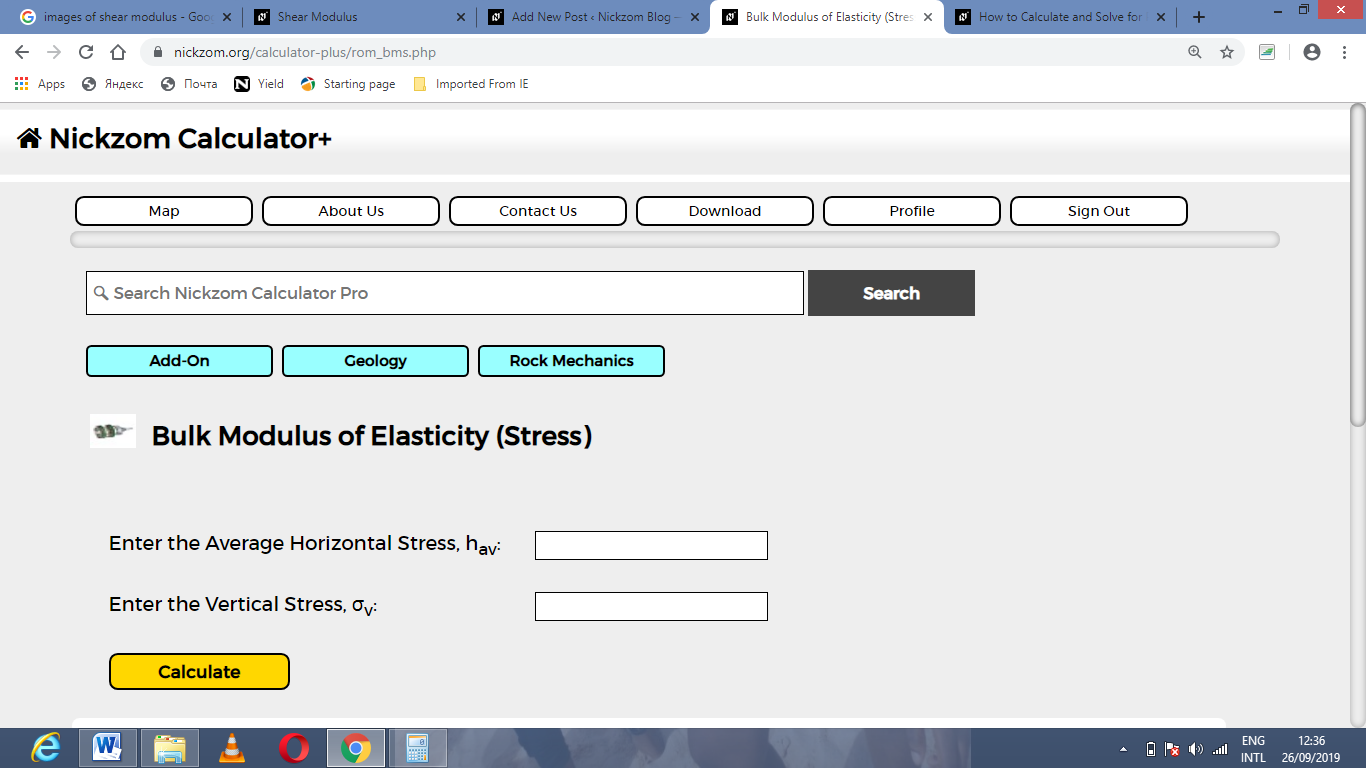Now, enter the values appropriately and accordingly for the parameters as required by the Average Horizontal Stress (hav) is 15 and Vertical Stress (σv) is 34.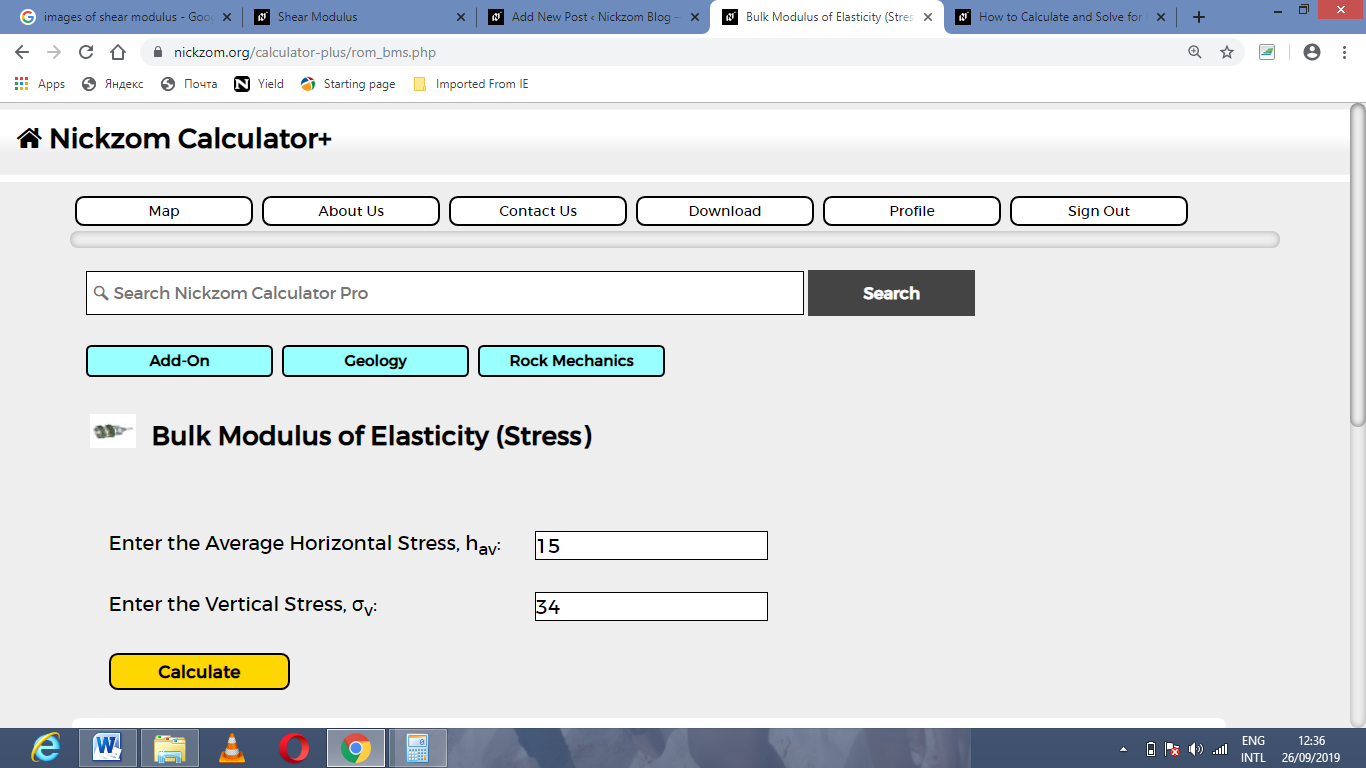Finally, Click on Calculate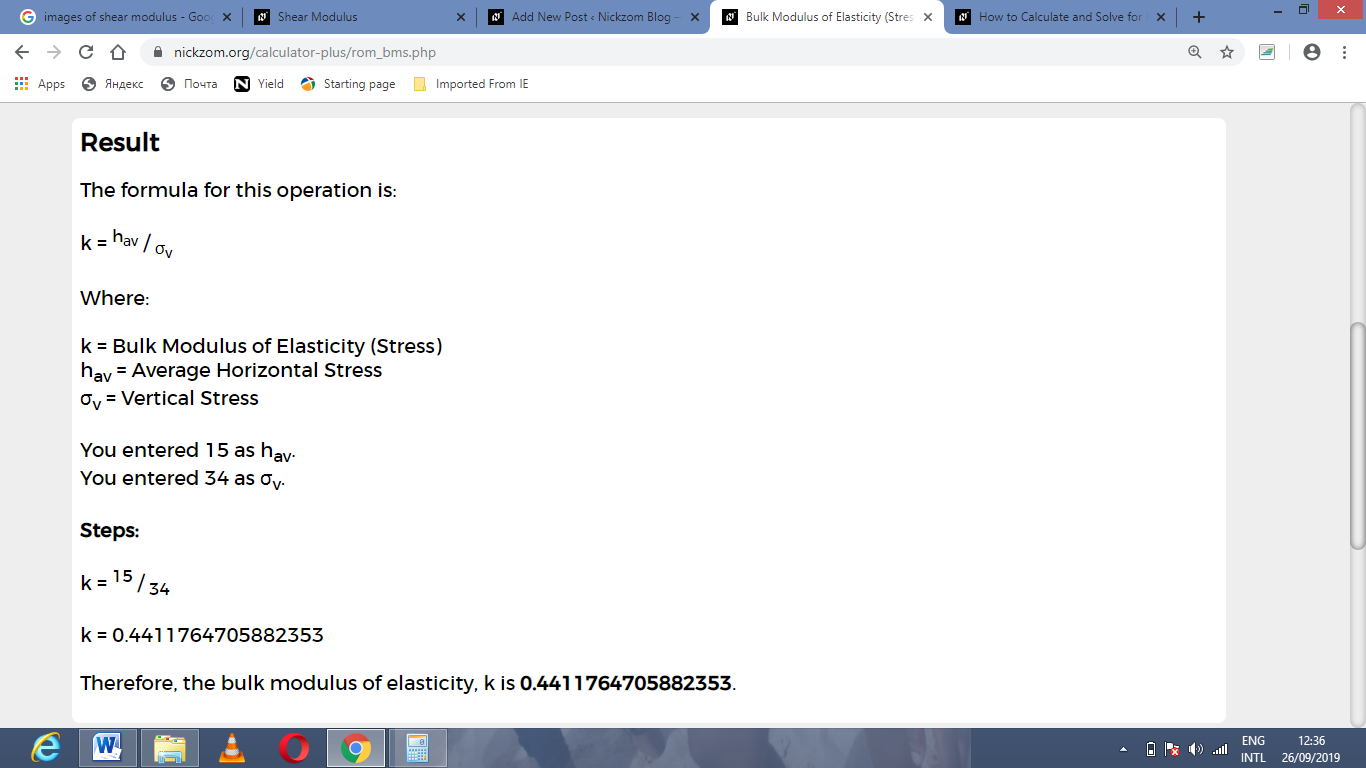As you can see from the screenshot above, Nickzom Calculator– The Calculator Encyclopedia solves for the bulk modulus of elasticity (stress) and presents the formula, workings and steps too.